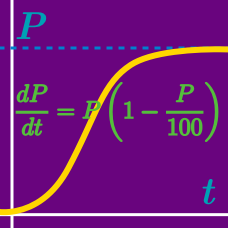Calculus

# Differential Equations - Modeling

Let $P(t)$ represent the amount of chemical a factory produces as a function of time $t$ (in hours). The rate of change of chemical production satisfies the differential equation $P'(t) = -\ln 3 \times P(t) \left( 1 - \frac{P(t)}{3}\right).$ If the factory alarm is raised when chemical production exceeds $4$ in $4$ hours, which of the following inequalities represents the maximum initial amount $P(0)$ of chemical that guarantees the alarm will not be raised?

Suppose the number of cells in a culture is approximated by $P(t)$ at time $t.$ If $P(t)$ satisfies the differential equation $P'(t) = \ln 2 \times P(t) \left( 1 - \frac{P(t)}{12}\right)$ and the initial number of cells is $P(0)=9,$ what is the approximation for the number of cells in the culture at time $t=3?$

Suppose the ratio of healthy cells to infected cells in a petri dish at time $t$ is represented by $P(t)$. If $P(t)$ satisfies the logistic differential equation $P'(t) = -\ln 5 \times P(t) \left( 1 - \frac{P(t)}{7}\right)$ and $P(0)= 2$, what is the value of $P(3)?$

Suppose the percentage of people surviving a dangerous virus at time $t$ is approximated by $P(t)$, where $P(0)=100.$ If $P(t)$ satisfies the logistic differential equation $P'(t) = 6 \times P(t) \left( 1 - \frac{P(t)}{20}\right),$ at what value of $t$ is the survival rate $75$%?

Suppose the population in a park at time $t$ is given by $P(t)$, where $P'(t) = -0.5 \times P(t) \left( 1 - \frac{P(t)}{5}\right).$ If $P(0) = 12,$ at what time $t$ does the population in the park reach $16?$

×